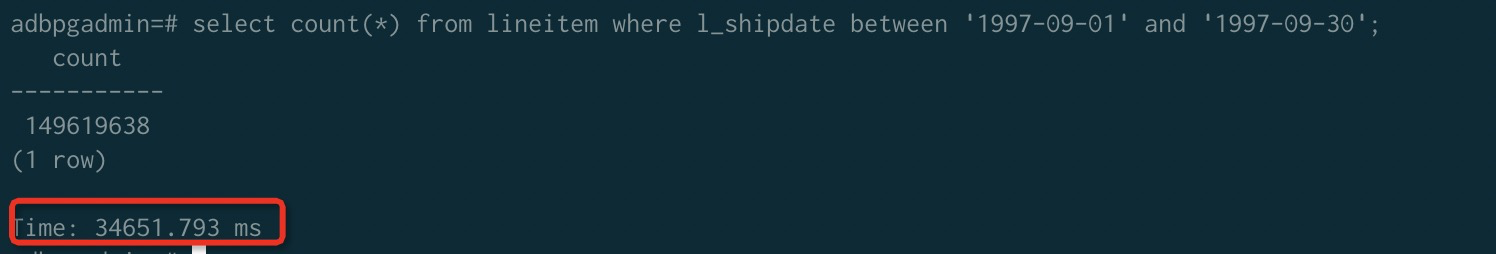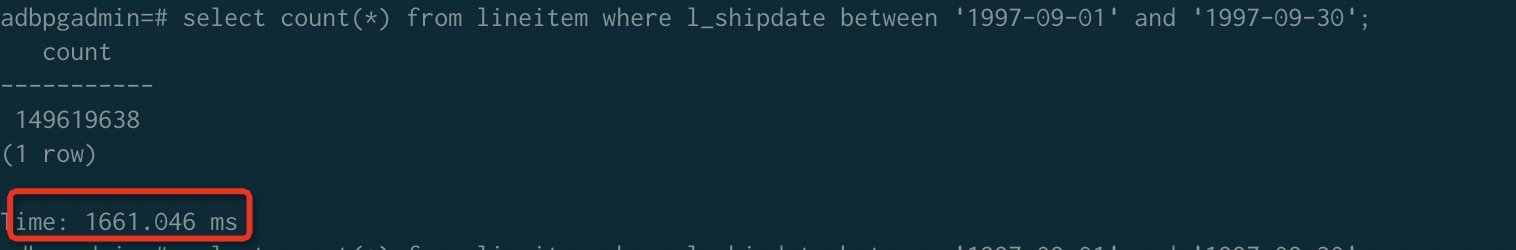• 存储预留模式：数据库内核版本为20200826版本之后的新建实例。
• 存储弹性模式：数据库内核版本为20200906版本之后的新建实例。

## 背景信息

• 组合排序：适用于限定条件是查询的前缀子集或者完全包含排序键，更适合于查询包含首列限定条件的情况。
• 多维排序：给每一个排序键分配相同的权重，更适合于查询条件包含任意限定条件子集的场景。

1. 创建一个32节点的实例。
2. 对Lineitem写入130亿行记录。
3. 查询1997-09-01到1997-09-30的数据。
• 数据未按照l_shipdate排序。• 数据按照l_shipdate排序。## 创建表时定义排序键

``create table test(date text, time text, open float, high float, low float, volume int) with(APPENDONLY=true,ORIENTATION=column) ORDER BY (volume);``

``````CREATE [[GLOBAL | LOCAL] {TEMPORARY | TEMP}] TABLE table_name (
[ { column_name data_type  ...} ]
)
[ DISTRIBUTED BY (column, [ ... ] ) | DISTRIBUTED RANDOMLY ]
[ ORDER BY (column, [ ... ] )]``````

## 对表进行排序

``SORT [tablename]``

``VACUUM SORT ONLY [tablename]``

``MULTISORT [tablename]``

``VACUUM REINDEX [tablename]``

## 修改排序键

``ALTER [[GLOBAL | LOCAL] {TEMPORARY | TEMP}] TABLE table_name SET ORDER BY (column, [ ... ] )``

``ALTER TABLE test SET ORDER BY(high,low);``

``ALTER TABLE test SET SORTKEY(high,low);``

## 组合排序和多维排序的性能对比

1. 创建测试表并设置表的排序键。
2. 写入测试数据。
3. 分别对这张表做组合排序和多维排序。
4. 对比同样的SQL场景，组合排序和多维排序的点查性能。
5. 对比同样的SQL场景，组合排序和多维排序的范围查询性能。

``````CREATE TABLE test(id int, num1 int, num2 int, value varchar)
with(APPENDONLY=TRUE, ORIENTATION=column)
DISTRIBUTED BY(id)
ORDER BY(id, num1, num2);

CREATE TABLE test_multi(id int, num1 int, num2 int, value varchar)
with(APPENDONLY=TRUE, ORIENTATION=column)
DISTRIBUTED BY(id)
ORDER BY(id, num1, num2);``````

``````INSERT INTO test(id, num1, num2, value) select g,
(random()*10000000)::int,
(random()*10000000)::int,
(array['foo', 'bar', 'baz', 'quux', 'boy', 'girl', 'mouse', 'child', 'phone'])[floor(random() * 10 +1)]
FROM generate_series(1, 10000000) as g;

INSERT INTO test_multi SELECT * FROM test;

count
----------
10000000
(1 row)

count
----------
10000000
(1 row)``````

``````SORT test;
MULTISORT test_multi;``````

• 包含首列排序键限定条件。
``````-- Q1 包含首列限定条件
select * from test where id = 100000;
select * from test_multi where id = 100000;``````
• 包含第二列限定条件。
``````-- Q2 包含第二列限定条件
select * from test where num1 = 8766963;
select * from test_multi where num1 = 8766963;``````
• 包含二三列限定条件。
``````-- Q3 包含二三列限定条件
select * from test where num1 = 100000 and num2=2904114;
select * from test_multi where num1 = 100000 and num2=2904114;``````

• 包含首列排序键限定条件。
``````-- Q1 包含首列限定条件
select count(*) from test where id>5000 and id < 100000;
select count(*) from test_multi where id>5000 and id < 100000;``````
• 包含第二列限定条件。
``````-- Q2 包含第二列限定条件
select count(*) from test where num1 >5000 and num1 <100000;
select count(*) from test_multi where num1 >5000 and num1 <100000;``````
• 包含二三列限定条件。
``````-- Q3 包含二三列限定条件
select count(*) from test where num1 >5000 and num1 <100000; and num2 < 100000;
select count(*) from test_multi where num1 >5000 and num1 <100000 and num2 < 100000;``````

• 对于Q1场景，由于包含排序键的首列，所以组合排序的效果非常好，而多维排序则会相对性能弱一些。
• 对于Q2场景，由于不包含排序键的首列，组合排序基本上失效了，而多维排序依然能维持比较稳定的性能提升。
• 对于Q3场景，由于不包含排序键的首列，组合排序依然起不到很好的效果，并且由于比较条件的增加，需要额外的比较开销，时间更长，而多维排序表现出更好的性能，这是因为在查询时，限定条件包含的多维排序键越多，性能越好。